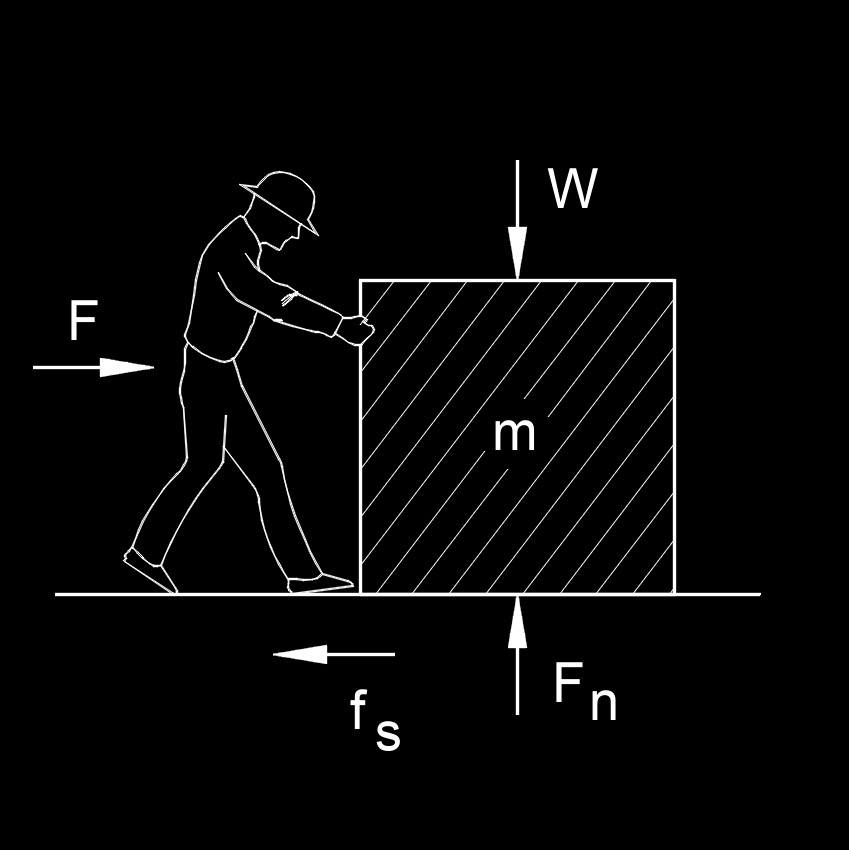# Static Friction

Written by Jerry Ratzlaff on . Posted in Classical MechanicsStatic friction, abbreviated as $$f_s$$, is the force that resists relative movement and keeps objects at rest.  Static friction happens between zero and the smallest force needed to start the motion of an object.  In order for an object to move the static friction must be overcome, when this happens you experience kinetic friction

## Formulas that use Static Friction

 $$\large{ f_s = \mu_s \; F_n }$$

### Where:

$$\large{ f_s }$$ = static friction

$$\large{ \mu _s }$$  (Greek symbol mu) = static friction coefficient

$$\large{ F_n }$$ = normal force